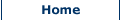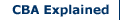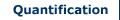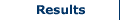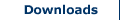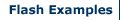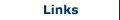Cost effectiveness analysis (CEA)

Cost Effectiveness Analysis (CEA) is an alternative form of CBA. It assesses the net cost of a project or service relative to the outcomes (benefits) generated. CEA is used where the need for a project has already been established, but uncertainty remains over the best method for achieving it. It is primarily used in health and defence policy as it, unlike more standard forms of CBA, bypasses three constraints (see Boardman et al, 2008, 463):

1. It does not require all costs and benefits to be put into monetary terms.
2. It acknowledges that a measure may not represent all of the benefit that is accrued.
3. It may be analysing intermediate goods (outcomes may not be clear).

As such it does not constrain the researcher into having to apply monetary values to certain variables. For example, the development of a new hospital would likely result in a number of saved lives in an area, but whereas standard forms of CBA would require a monetary value be placed on the value of a life saved, CEA does not require this. This makes it particularly suitable for health policy.

CEA considers alternatives in reference to the ratio between the costs associated with each alternative and a single quantified, but not monetary, effectiveness measure. This represents a complication of CEA as costs are represented by monetary values, while effectiveness may be measured in terms of saved lives, time savings or other similar quantifiable measures. For this reason in CEA a ratio is calculated. Two forms of ratio can be expressed:

1. Cost-Effectiveness Ratio: dividing costs of an alternative by the measure of effectiveness.
2. Effectiveness-Cost Ratio: dividing effectiveness measured by costs of alternative.

Using these ratios the researcher can compare two project alternatives as follows: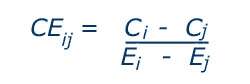Where,
Ci = Costs of alternative i
Cj = Costs of alternative j
Ei = Effectiveness units of alternative i
Ej = Effectiveness units of alternative j

Example

Consider a project for which there are four alternatives. The project involves adding a safety barrier to the centre reservation of a stretch of a major road. The cost per mile of the barrier differs depending on the quality of the material used. Scheme A uses a reinforced wooded barrier. Scheme B uses iron, C uses steel, and D uses concrete reinforced with steel. The costs and effectiveness in terms of lives saved are shown in the table below.

 Scheme Cost (£) E (lives saved per annum) C/E ΔC ΔE ΔC/ΔE A 9,000 5 1,800 - - - B 15,000 8 1,875 6,000 3 2,000 C 20,000 11 1,818 5,000 3 1,667 D 30,000 12 2,500 10,000 1 10,000

Simply calculating the ratio of costs to effectiveness (C/E) of each scheme suggests scheme A to be the most cost effective of the alternatives considered, as it is estimated it will result in one life being saved for every £1,800 spent. However, this simple approach does not consider the incremental difference provided by each scheme. This is especially important considering that scheme A is estimated to result in five lives being saved, while scheme D will potentially save 12 lives per year.

Instead the incremental cost-effectiveness ratio can be calculated, as in the table. This calculates the cost effectiveness of each alternative relative to the change in costs and effectiveness compared with the previous, cheaper, alternative. In the above example, it can be seen that the lowest cost associated with a incremental change in effectiveness, that is an additional life saved, is associated with scheme C. Scheme C costs £5,000 more than Scheme B (and £11,000 more than scheme A), but it results in a further three lives being saved each year compared with scheme B. This gives an incremental cost effectiveness ratio in comparison to scheme B of £1,667. In this example, the choice of scheme would differ depending on whether a simple cost-effectiveness or incremental cost-effectiveness ratio was calculated. In the prior case scheme A would be chosen, but this ignores the added effectives, in terms of lives saved, that is achieved by spending additional funds on scheme C.

Criticisms of CEA

The key criticism associated with CEA is in the use of a ratio measure. In CEA the prediction of costs and effectiveness, even more than in other forms of CBA, can result in incorrect outcomes due to the use of a ratio measure.

For this reason sensitivity analysis can be used by the researcher, as with other forms of CBA, to test the distribution of the cost effectiveness ratio relative to the normal distribution. This is sometimes referred to as a Monte Carlo Analysis (see Boardman et al, 2008, 470-473).

Search CBA Builder:

•